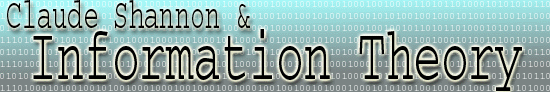Noise If entropy is a measure of information   in a source, then in a channel entropy is a measure of lost information. Any uncertainty in a channel concerning information is bad. In this way, let us define noise as "negative information." Physically, noise can be caused by random thermal motion or random voltage fluctuations. However, in information theory, it is convenient to view a noisy channel as a black box which garbles any signal sent through it. Noise plays a crucial role in determining the capacity, or bits of information per second, that get through a communications channel. The amount of noise in the channel will determine whether it is possible to transmit information at channel capacity with a vanishing error rate. Furthermore, if information must be reliably transmitted, noise necessitates redundancy in any code for error checking. Like information, noise is viewed stochastically in Shannon's information theory. Noise is the entropy associated with the probability that one symbol will change to another symbol while being transmitted across a channel.  A general formula for noise is obtained by essentially measuring the decrease in uncertainty that occurs when a signal is received. Say we recieve the symbol X. What does this tell us about what was sent? If the receipt tells us nothing about what was sent, then the channel is essentially useless to send information; it's noise is at a maximum. Although the mathematics of deriving a general formula for calculating the entropy due to noise is rather complicated, a general explanation follows. Let p(i) be the probability of symbol si being generated by the transmitter. Let p(j) be probability that symbol sj is received by the receiver. Now let p(i,j) be the probability that si is sent, and sj is received. The ratio of p(i,j)/(p(i) * p(j)) gives us a means to measure entropy. It measures how much the symbol sj depends on what is sent (si). If the receipt of sj makes it more likely that si was sent, then the ratio will be greater than 1. A ratio of 1 indicates that the reciept of sj tells us nothing  we didn't know before concerning whether si was sent. This ratio is used to calculate the entropy associated with the noise level of the channel.